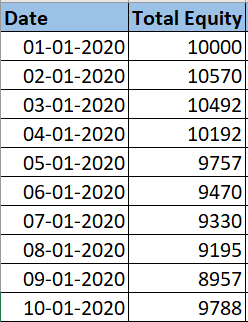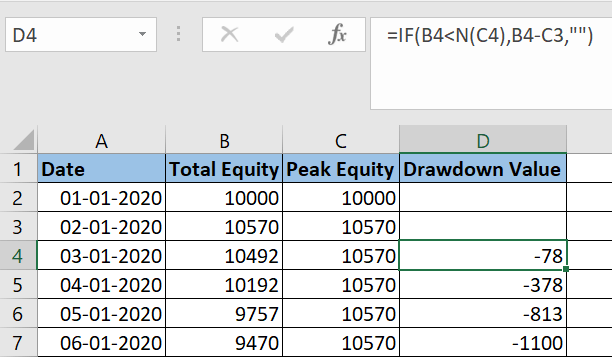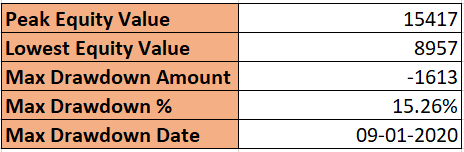# How to calculate Drawdown in an Excel sheet?

Drawdown is one of the most important measures for evaluating trading systems. A drawdown measures the peak to trough decline of your equity value during a specific period of time. In this post, we’ll learn how to calculate drawdown in an excel sheet. Although this metric is readily available in most of the modern backtesting tools, yet having it in Excel is going to add a lot of values for newbies.

## Understanding Drawdowns

Usually measured in % terms, drawdown tells you how much your equity declined from its peak value.

For example – You buy one stock at \$100 and your first trade gave you a 20% profit. So your equity value would be \$120. Now the stock drops from 120 to 110, that’s \$10 off the highs and therefore a \$10 or 8.3% drawdown from the so-called peak.Any trading system or strategy is prone to drawdowns, you literally cannot have a system with zero drawdowns. The real deal is how to minimize these drawdowns, which is a different topic altogether and we’ll eventually cover it in another blog post.

## Calculate Drawdown in an Excel Sheet

Drawdown calculation in the Excel sheet is pretty simple and can be achieved through some simple mathematical formulas.

First of all, you need to list down your total equity(capital) arranged in order of dates. Suppose you put this information in columns A and BThen, in column C you need to calculate ‘Peak Equity’ value. It is simply the max of current equity and previous peak value.Column D will contain the drawdown value. It is the difference between current equity and peak equity.And column E will represent Drawdown %, which is nothing but the drawdown expressed in % of peak equity.Once you are done with these calculations, you can report the final statistics using Excel min/max function. Download the sample excel sheet to understand these functions